# PCA降维算法（内含PCA可视化迷你案例+PCA人脸识别降维案例+PCA逆转降噪案例） 原创1512-高同学

# 1、sklearn中的降维算法

PCA使用的信息量衡量指标，就是样本方差，又称可解释性方 差，方差越大，特征所带的信息量越多。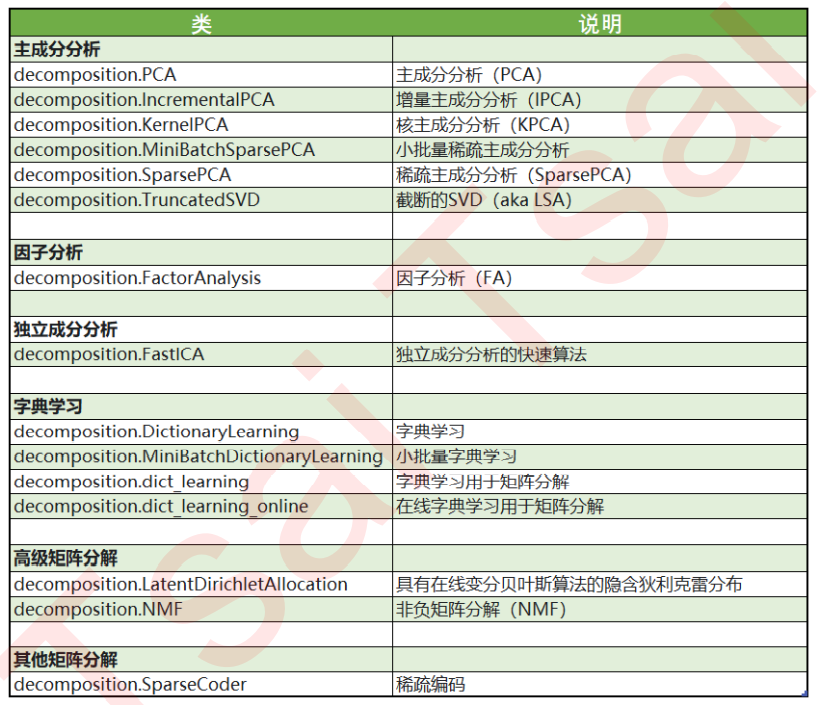## （1）PCA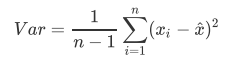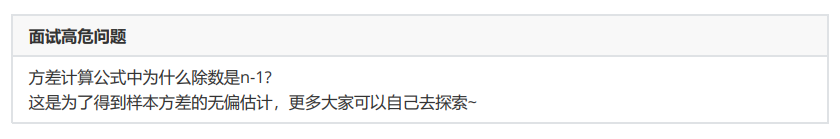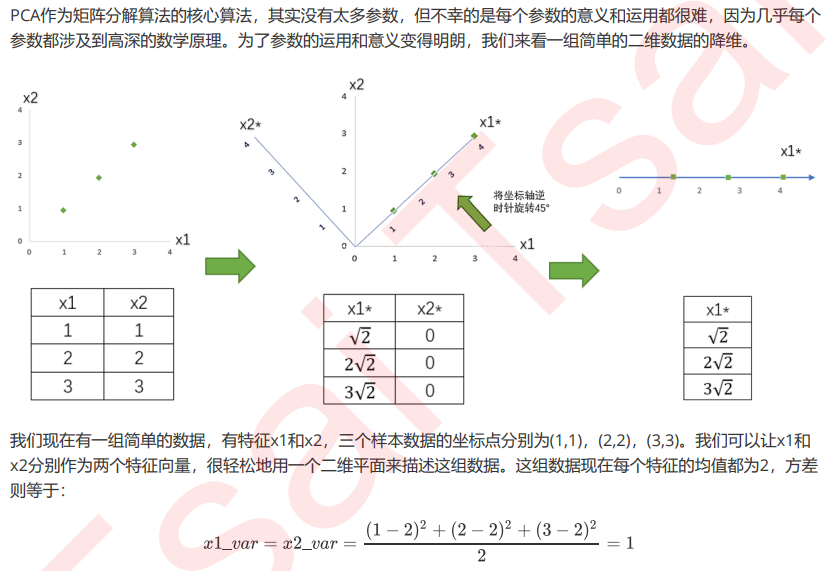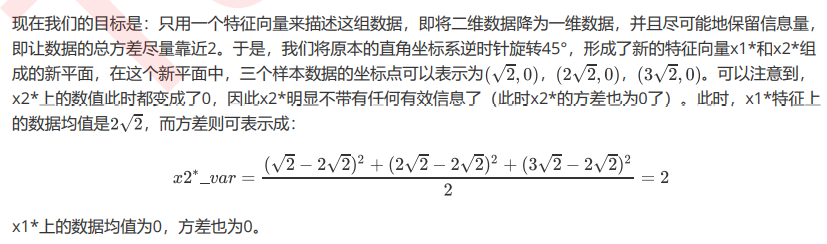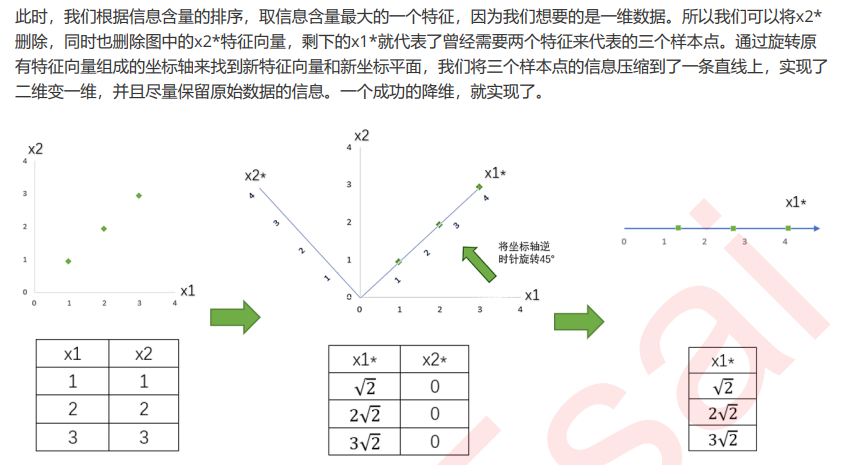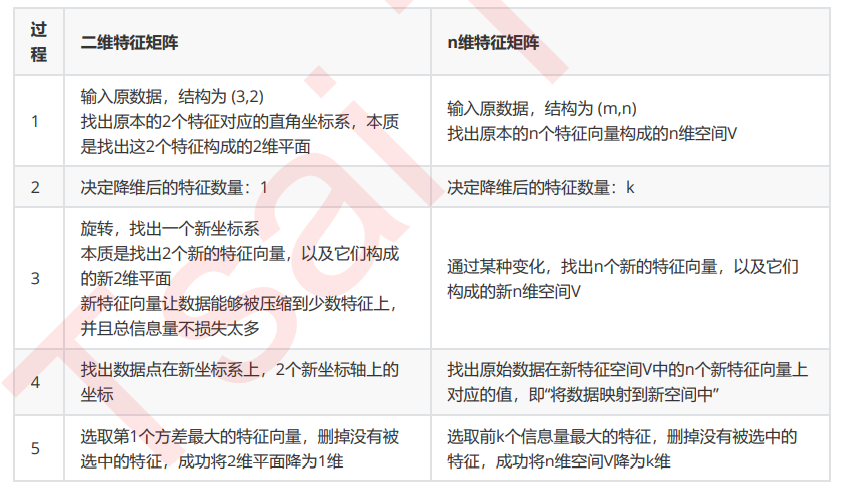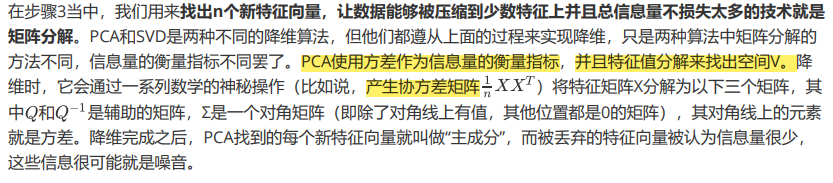## （2）SVD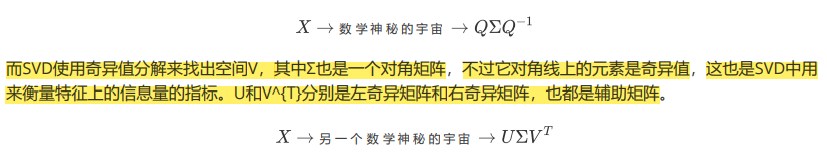## （3）思考

PCA和特征选择技术都是特征工程的一部分，它们有什么不同？

# 2、重要参数n_components

n_components是我们降维后需要的维度，即降维后需要保留的特征数量，降维流程中第二步里需要确认的k值， 一般输入[0, min(X.shape)]范围中的整数。K是一个需要我们人为去确认的超参数，并且我们设定的数字会影响到模型的表现。就达不到降维的效果，如果留下的特征太少，那新特征向量可能无法容纳原始数据集中的大部分信息，因此，n_components既不能太大也不能太小。那怎么办呢？

from sklearn.decomposition import PCA
from matplotlib import pyplot as plt
import pandas as pd
import numpy as np

#print(iris)

x = iris.data
y = iris.target
#print(x)
#print(y)
#print(pd.DataFrame(x))
#可以了解到鸢尾花数据集一共有4个特征

pca = PCA(n_components=2) #实例化
pca = pca.fit(x)          #弥合模型
result = pca.transform(x) #获取新矩阵
#print(result)   #新矩阵只有两个特征值

x_dr = PCA(n_components=2).fit_transform(x)
#print(x_dr)    #这两种方法结果是一样的

#可视化，将三种鸢尾花的数据分布显示在二维平面坐标系中，对应的两个字符欧标应该是三种鸢尾花降维之后的坐标位置
#选取每一个种类的特征值
#print(x_dr[y == 0, 0]) #选取标签为1的第一列值，第二列也是一个意思的
plt.figure()
plt.scatter(x_dr[y == 0, 0], x_dr[y == 0, 1], color='red', label=iris.target_names)
plt.scatter(x_dr[y == 1, 0], x_dr[y == 1, 1], color='black', label=iris.target_names)
plt.scatter(x_dr[y == 2, 0], x_dr[y == 2, 1], color='orange', label=iris.target_names)
plt.title('pca of IRIS dataset')
plt.legend()
plt.show()

#这两种方法效果是一样的，一个不写循环，一个写循环
colors = ['red', 'black', 'yellow']
for i in range(3):
plt.scatter(x_dr[y == i, 0], x_dr[y == i, 1], alpha=0.7, color=colors[i],label=iris.target_names[i])

plt.legend()
plt.title('PCA of IRIS dataset')
plt.show()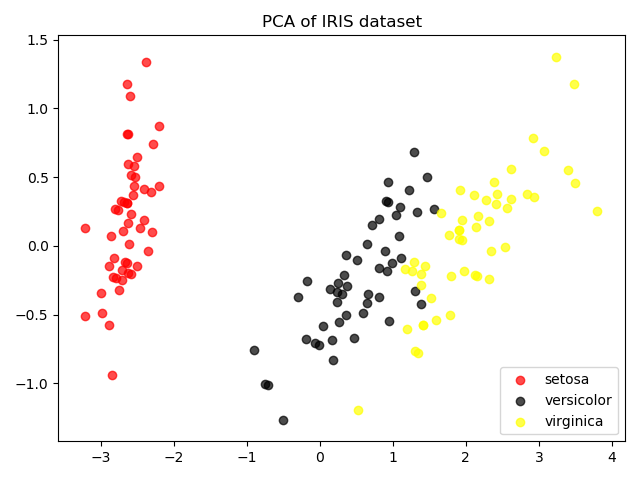#探索降维后的数据
print(pca.explained_variance_ )
#查看降维后每个特征向量上所带的信息大小（可解释方差的大小）
#[4.22824171 0.24267075]  #就是每个新特征的方差值

print(pca.explained_variance_ratio_)  #查看降维后每个新特征向量所占的信息量占原始数据总信息量的百分比
#[0.92461872 0.05306648]

print(pca.explained_variance_ratio_.sum())
#0.9776852063187949

pca_line = PCA().fit(x)  #当不填写n_compents的时候，会计算每个特征向量的贡献值
plt.plot([1, 2, 3, 4], np.cumsum(pca_line.explained_variance_ratio_))  #np.cumsum将每个特征信息比例进行累加
plt.xticks([1, 2, 3, 4])  #这是为了限制坐标轴显示为整数
plt.xlabel('number of components after dimension reduction')
plt.ylabel('cumulative explained variance ratio')
plt.show()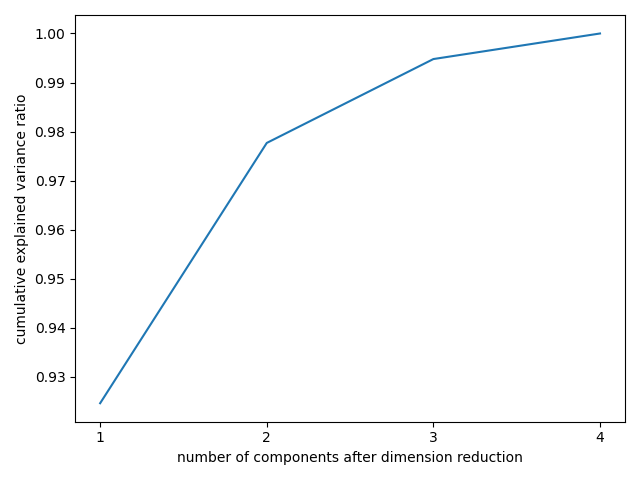pca = PCA(n_components='mle')
#让pca进行选择降维到几维
pca_mle = pca.fit(x)
x_mle = pca_mle.transform(x)


pca_f = PCA(n_components=0.97, svd_solver='full')
pca_f.fit(x)
x_f = pca_f.transform(x)
#print(x_f)

print(pca_f.explained_variance_ratio_)
#[0.92461872 0.05306648]


# 3、PCA中的SVD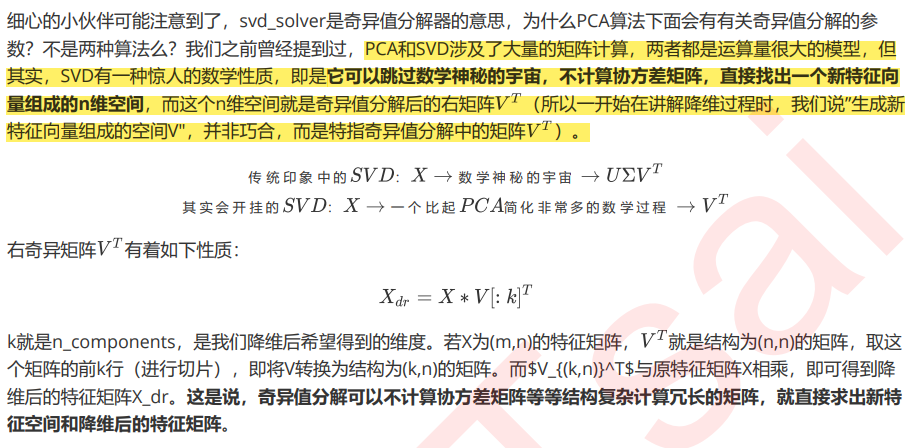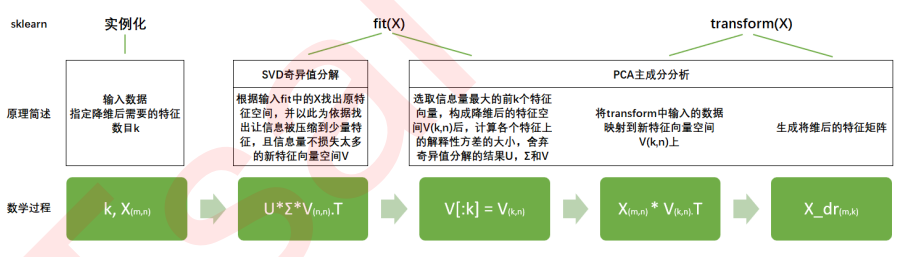“auto”：基于X.shape和n_components的默认策略来选择分解器：如果输入数据的尺寸大于500×500且要提 取的特征数小于数据最小维度min(X.shape)的80％，就启用效率更高的”randomized“方法。否则，精确完整 的SVD将被计算，截断将会在矩阵被分解完成后有选择地发生。

“full”：从scipy.linalg.svd中调用标准的LAPACK分解器来生成精确完整的SVD，适合数据量比较适中，计算时 间充足的情况，生成的精确完整的SVD的结构为：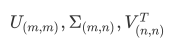“arpack”：从scipy.sparse.linalg.svds调用ARPACK分解器来运行截断奇异值分解(SVD truncated)，分解时就将特征数量降到n_components中输入的数值k，可以加快运算速度，适合特征矩阵很大的时候，但一般用于 特征矩阵为稀疏矩阵的情况，此过程包含一定的随机性。截断后的SVD分解出的结构为：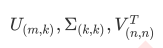“randomized”，通过Halko等人的随机方法进行随机SVD。在"full"方法中，分解器会根据原始数据和输入的 n_components值去计算和寻找符合需求的新特征向量，但是在"randomized"方法中，分解器会先生成多个随机向量，然后一一去检测这些随机向量中是否有任何一个符合我们的分解需求，如果符合，就保留这个随 机向量，并基于这个随机向量来构建后续的向量空间。这个方法已经被Halko等人证明，比"full"模式下计算快 很多，并且还能够保证模型运行效果。适合特征矩阵巨大，计算量庞大的情况。

from sklearn.decomposition import PCA
from sklearn.datasets import fetch_lfw_people
from matplotlib import pyplot as plt
import numpy as np
import pandas as pd

faces = fetch_lfw_people(min_faces_per_person=60)  #实例化
#print(faces.images.shape)  #(1348, 62, 47)
#print(faces.data.shape)  #(1348, 2914)  2914 = 62 * 47
x = faces.data #设置特征矩阵

#画图，将原特征矩阵进行可视化
fig, axes = plt.subplots(4, 5, figsize=(8, 4), subplot_kw={'xticks': [], 'yticks': []}) #不要显示坐标轴
#print(fig)
#print(axes) ##不难发现，axes中的一个对象对应fig中的一个空格
#print(axes.shape)  #(4, 5) 四行五列图片

#二维结构，可以有两种循环方式，一种是使用索引，循环一次同时生成一列上的三个图
#另一种是把数据拉成一维，循环一次只生成一个图
#在这里，究竟使用哪一种循环方式，是要看我们要画的图的信息，储存在一个怎样的结构里
#我们使用 子图对象.imshow 来将图像填充到空白画布上
#而imshow要求的数据格式必须是一个(m,n)格式的矩阵，即每个数据都是一张单独的图
#因此我们需要遍历的是faces.images，其结构是(1277, 62, 47)
#要从一个数据集中取出24个图，明显是一次性的循环切片[i,:,:]来得便利
#因此我们要把axes的结构拉成一维来循环

#print(enumerate(axes.flat))
#填充图像
for i, ax in enumerate(axes.flat):
ax.imshow(faces.images[i, :, :], cmap='gray')#选择色彩模式
#plt.show()
#print(fig)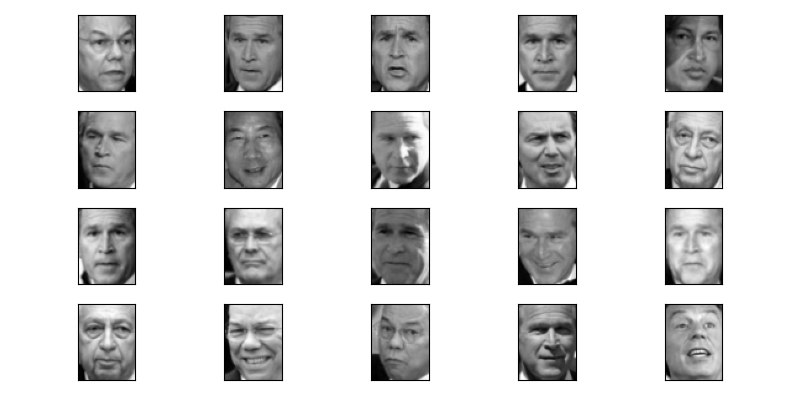#建模降维，提取新特征空间矩阵
pca = PCA(150).fit(x) #将data原来的2914维降到150维
v = pca.components_
#print(v.shape)  #(150, 2914) （新特征维度，原特征维度）

#print(v[0, :].reshape(62, 47))
'''
[[-0.00579708 -0.00595363 -0.00615767 ... -0.00813068 -0.00789127
-0.00791125]
[-0.00586497 -0.00604798 -0.00647989 ... -0.00849601 -0.00826257
-0.00840366]
[-0.00622997 -0.00658633 -0.00714348 ... -0.00897475 -0.0085009
-0.0089165 ]
...
[-0.00270353 -0.00276129 -0.00370097 ... -0.01046781 -0.00896865
-0.00820496]
[-0.00298345 -0.003292   -0.00394306 ... -0.01013859 -0.00875235
-0.00805416]
[-0.00329591 -0.00362476 -0.00457148 ... -0.01000113 -0.00901094
-0.00813919]]
'''

#print(v[0, :]) #取降维后的像素，新的特征将轴进行了变换，我们想看看变换后的图像是什么样的
'''
[-0.00579718 -0.00595365 -0.00615768 ... -0.01000111 -0.00901092
-0.00813918]
'''

#将新特征空间矩阵可视化
fig, axes = plt.subplots(3, 8, figsize=(8, 4), subplot_kw={'xticks': [], 'yticks': []})

for i, ax in enumerate(axes.flat):
ax.imshow(v[i, :].reshape(62, 47), cmap='gray')
plt.show()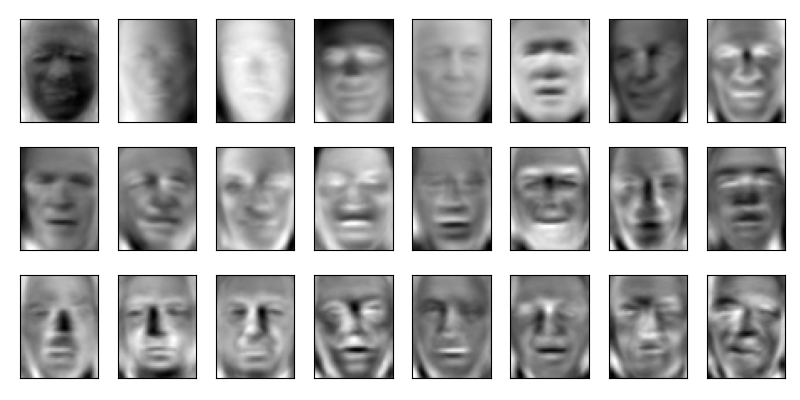# 4、重要接口inverse_transform

from sklearn.datasets import fetch_lfw_people
from sklearn.decomposition import PCA
import numpy as np
import pandas as pd
from matplotlib import pyplot as plt

faces = fetch_lfw_people(min_faces_per_person=60)
x = faces.data
#print(x)
#print(x.shape) #(1348, 2914)

pca = PCA(150)
x_dr = pca.fit_transform(x)

#print(x_dr)
#print(x_dr.shape)

#print(x_dr)
x_inverse = pca.inverse_transform(x_dr)
#print(x_inverse)

fig, ax = plt.subplots(2, 10, figsize=(10, 2.5), subplot_kw={"xticks": [], "yticks": []})
#plt.show()
#print(ax)

for i in range(10):
ax[0, i].imshow(faces.images[i, :, :], cmap='binary_r')
ax[1, i].imshow(x_inverse[i].reshape(62, 47), cmap='binary_r')

plt.show()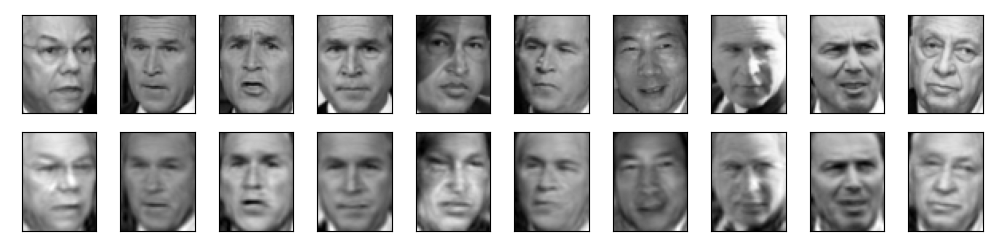from sklearn.datasets import load_digits
from sklearn.decomposition import PCA
import numpy as np
import pandas as pd
from matplotlib import pyplot as plt

#print(digits)

#print(digits.data.shape)#(1797, 64)
#print(digits.images.shape) #(1797, 8, 8)
#print(set(digits.target.tolist())) #{0, 1, 2, 3, 4, 5, 6, 7, 8, 9}
#是一个手写识别分类数据，标签是从0-9

def plot_digits(data):
fig, axes = plt.subplots(4, 10, figsize=(10, 4), subplot_kw={'xticks': [], 'yticks': []})
for i, ax in enumerate(axes.flat):
ax.imshow(data[i].reshape(8, 8), cmap="binary")
plt.show()

plot_digits(digits.data)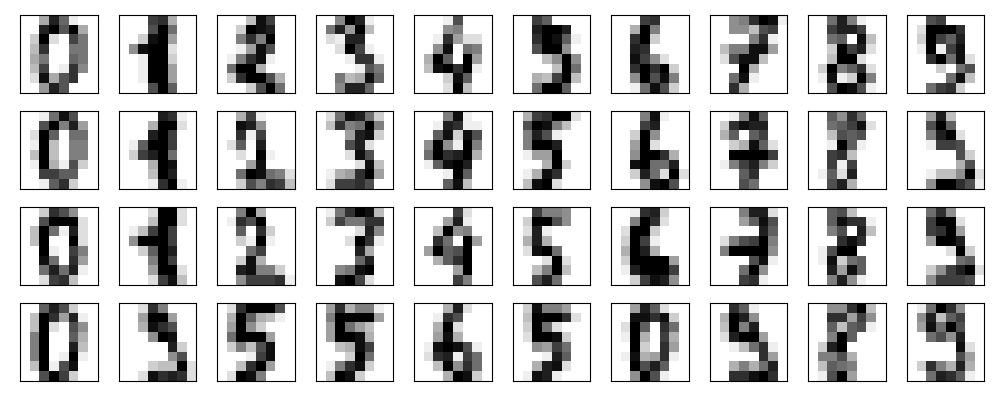rng = np.random.RandomState(42)
#给原始数据加上噪音

digits_noise = rng.normal(digits.data, 2)
#从原始数据集中取出来一个服从正太分布，方差为2的新数据集
#plot_digits(digits_noise)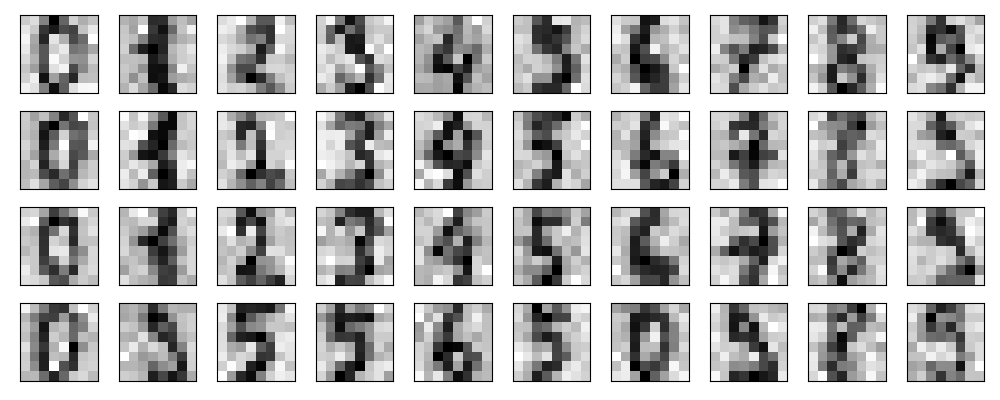pca = PCA(0.5, svd_solver='full').fit(digits_noise)
x_dr = pca.transform(digits_noise)
print(x_dr.shape)  #(1797, 6)

x_inverse = pca.inverse_transform(x_dr)
#plot_digits(x_inverse)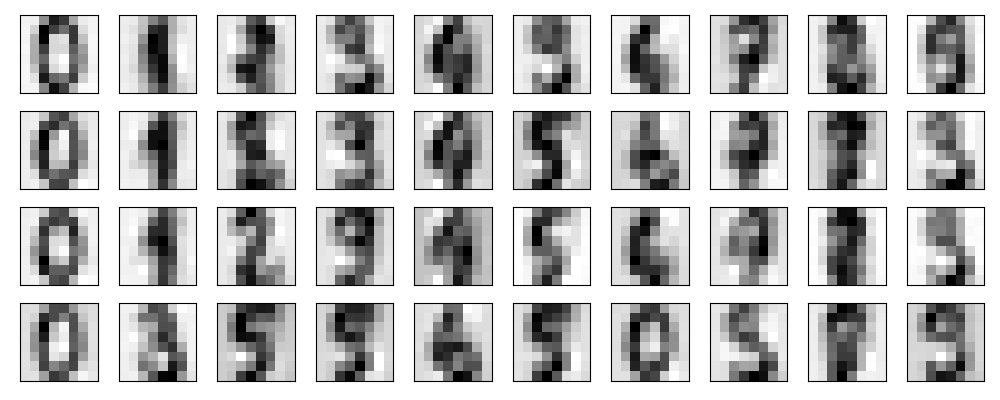# 5、重要接口，参数和属性总结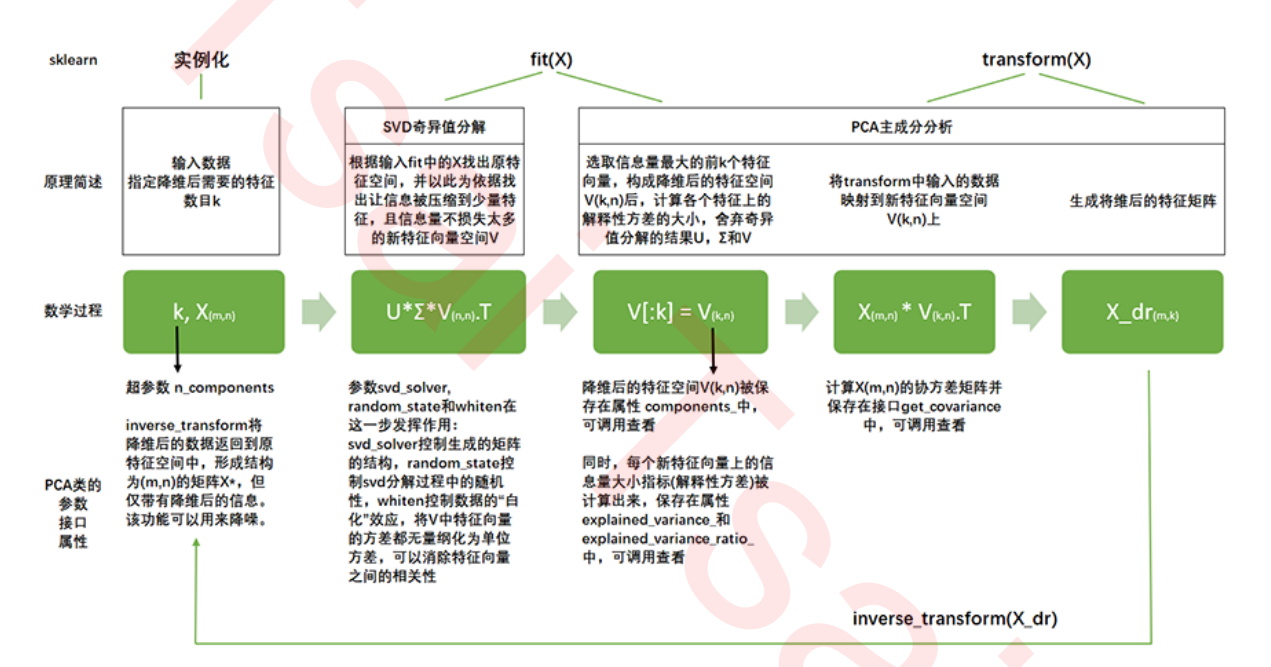# 6、案例：PCA对手写数字数据集的降维

from sklearn.decomposition import PCA
from sklearn.ensemble import RandomForestClassifier as RFC
from sklearn.neighbors import KNeighborsClassifier as KNN
from sklearn.model_selection import cross_val_score
import matplotlib.pyplot as plt
import numpy as np
import pandas as pd
import time

#print(data.shape)
#print(data.info())

x = data.iloc[:, 1:] #(42000, 785)
y = data.iloc[:, 0]

pca_line = PCA().fit(x)
plt.figure(figsize=(20, 5))
plt.plot(np.cumsum(pca_line.explained_variance_ratio_)) #将维度解释率累加
plt.xlabel('number of components after dimension reduction')
plt.ylabel('cumulative explained variance ratio')
#plt.show()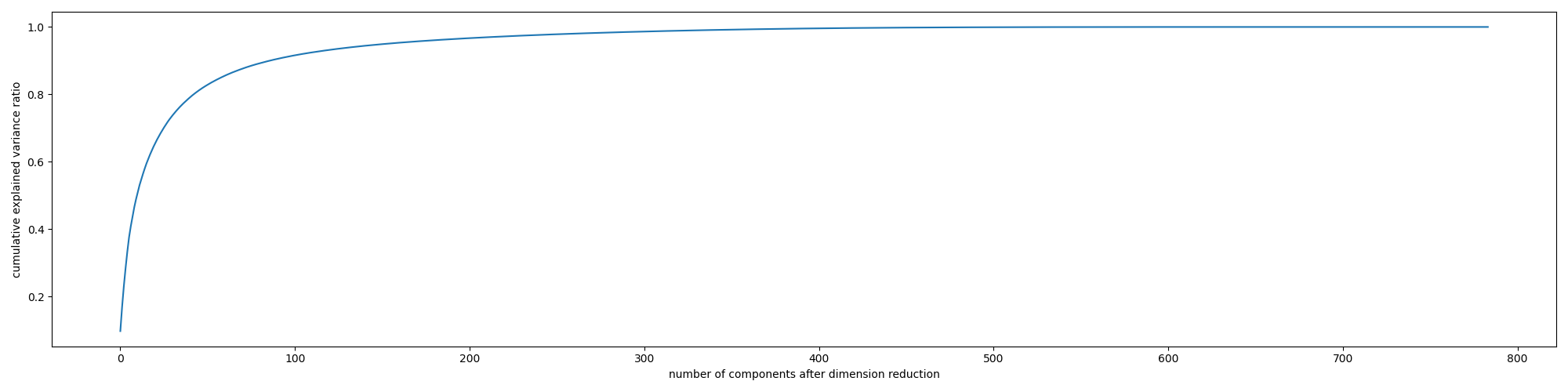scores = []
#继续缩小最佳维度的范围
for i in range(1, 101, 10):
x_dr = PCA(i).fit_transform(x)
once = cross_val_score(RFC(n_estimators=10, random_state=0), x_dr, y, cv=5).mean()
scores.append(once)
plt.figure(figsize=(10, 8))
plt.plot(range(1, 101, 10), scores)
plt.show()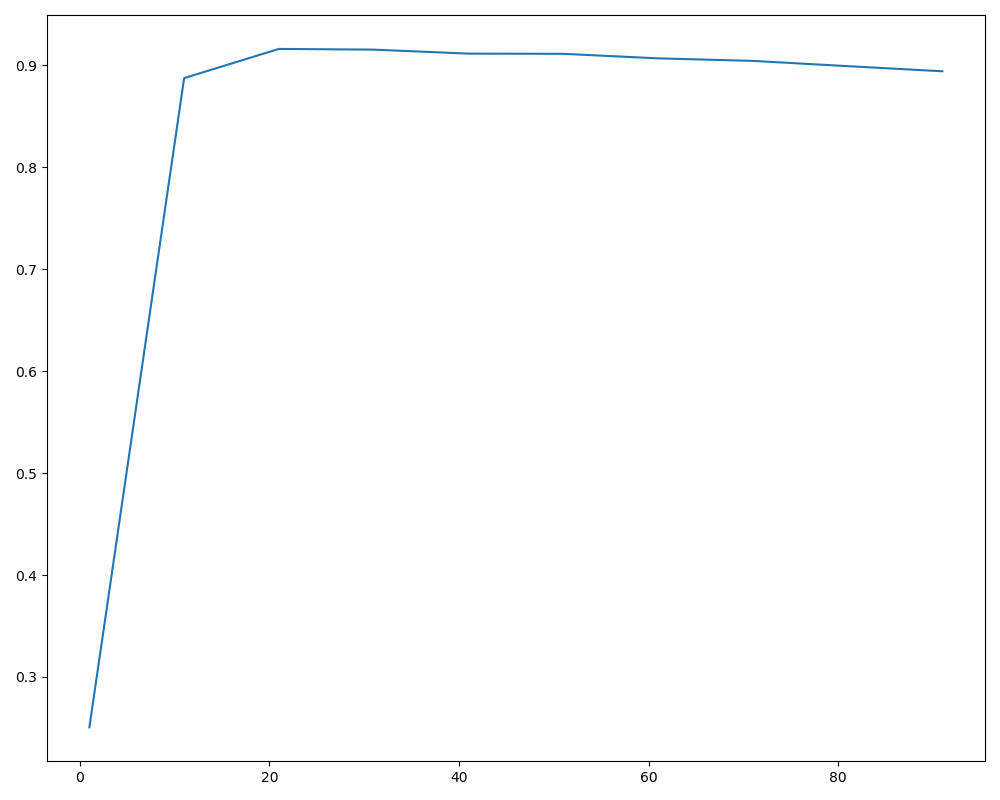start_time = time.time()
scores = []
#继续缩小最佳维度的范围
for i in range(15, 30):
x_dr = PCA(i).fit_transform(x)
once = cross_val_score(RFC(n_estimators=10, random_state=0), x_dr, y, cv=5).mean()
scores.append(once)
plt.figure(figsize=(10, 8))
plt.plot(range(15, 30), scores)
end_time = time.time()
print(end_time-start_time)
plt.show()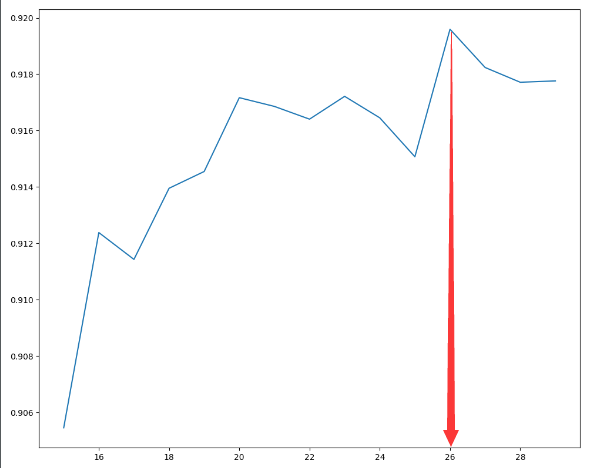x_dr = PCA(26).fit_transform(x)
score = cross_val_score(RFC(n_estimators=100, random_state=0), x_dr, y, cv=5).mean()
print(score)  #0.9466190476190477


x_dr = PCA(26).fit_transform(x)
score_knn = cross_val_score(KNN(), x_dr, y, cv=5).mean()
print(score_knn)  #0.9710238095238095


x_dr = PCA(26).fit_transform(x)
score_1 = []
for i in range(10):
once = cross_val_score(KNN(i+1), x_dr, y, cv=5).mean()
score_1.append(once)
plt.figure(figsize=(10, 8))
plt.plot(range(10), score_1)
plt.show()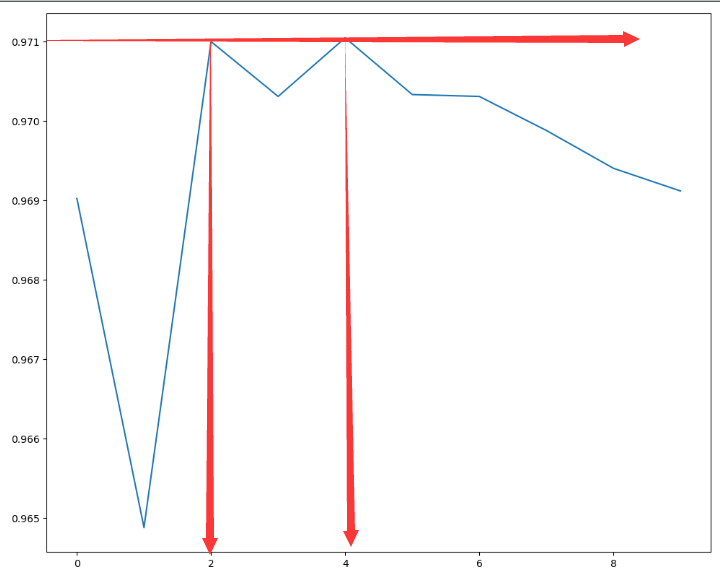x_dr = PCA(26).fit_transform(x)
score_knn = cross_val_score(KNN(3), x_dr, y, cv=5).mean()
print(score_knn)  #0.9708571428571429

x_dr = PCA(26).fit_transform(x)
score_r = cross_val_score(KNN(5), x_dr, y, cv=5).mean()
print(score_knn)  #0.9708571428571429


### 评论 抢沙发### 觉得文章有用就打赏一下文章作者

#### 支付宝扫一扫打赏#### 微信扫一扫打赏Vieu3.3主题Q Q 登 录